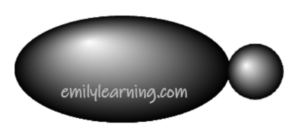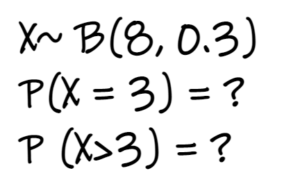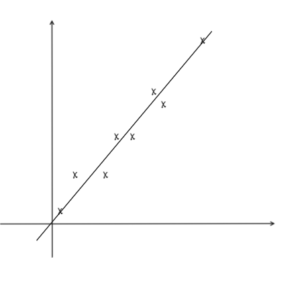# Blog## Orbital Hybridisation – sp³, sp², sp hybridisation

In this post, we’ll talk about sp³, sp² and sp orbital hybridisation. Also, I’ll show you an easy way to determine hybridisation.## How to find probabilities for binomial distribution with TI 84 graphic calculator

Let’s look at how to find probabilities for binomial distribution with Ti 84 CE plus graphic calculator, tested in statistics section.## Sampling Questions: A Level TYS Answers

Find the worked solutions for past A Level sampling questions, which are found in paper 2 of H2 Math papers, statistics section.## Hypothesis Testing Questions: A Level TYS Answers

Find the worked solutions for past A Level hypothesis testing questions, which are found in paper 2 of H2 Math papers, statistics section.## Regression and Correlation Questions: A Level TYS Answers

Find the worked solutions for past A Level regression and correlation questions, found in paper 2 of H2 Math papers, statistics section.## Binomial Distribution Questions: A Level TYS Answers

Find the worked solutions for past A Level binomial distribution questions. Binomial distribution questions are found in paper 2, statistics section of A Level Math.## Normal Distribution Questions: A Level TYS Answers

Find the worked solutions for past A Level normal distribution questions. Normal distribution questions are found in paper 2, statistics section.## Discrete Random Variable Questions: A Level TYS Answers

Find the worked solutions for past A Level discrete random variable questions, which are found in paper 2 of H2 Math papers, statistics section.## Probability Questions: A Level TYS Answers

Find the worked solutions for past A Level probability questions. Probability questions are found in paper 2.## Permutation and Combination Questions: A Level TYS Answers

Find the worked solutions for past A Level permutation and combination questions. Permutation and combination questions are found in paper 2.